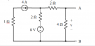# Nodal Analysis of a combo circuit

• Engineering
• SteliosVas

## Homework Statement

Attached is a image of the circuit that I am attempting to analyze:

KCL

## The Attempt at a Solution

So I know I should assign a place in the circuit where I should preform KCL after selecting a reference point.

I selected the reference point as the 2ohm resistor with the right hand side being V2 and the left as V1

Would the KCL at v1 be:

-4+(v1-8)/2+(v1-v2)/2 =0

and KCL at v2 be:

(v2-v1)/2 + v2/4 =0

But I didn't get the right answer. I have not sure now...

#### Attachments

•Screen Shot 2016-05-31 at 6.08.58 PM.png
3.3 KB · Views: 450
Hi SteliosVas. What you have written looks right. So try again solving these equations.

What are you given as the correct answer?

•donpacino